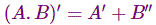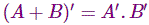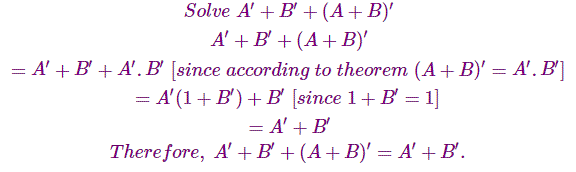# De Morgan’s Laws (Also Known As De Morgan’s Theorem

## What are De Morgan’s Laws?

De Morgan’s laws (also known as De Morgan’s theorem) are a pair of transformation rules used to simplify logical expressions in computer programs and digital circuit designs. They are named after their founder Augustus De Morgan, a 19th-century British mathematician. The rules allow the expression of conjunctions and disjunctions purely in terms of each other via negation.

Now I realise that sounds like some mathematical gibberish.

So let’s express these rules in English:

• the negation of a disjunction is the conjunction of the negations
• the negation of a conjunction is the disjunction of the negations

Or even more plainly as:

• the complement of the union of two sets is the same as the intersection of their complements
• the complement of the intersection of two sets is the same as the union of their complements

Combined with binary arithmetic—such as binary addition, binary subtraction, binary multiplication and binary division—De-Morgan’s Laws make it significantly easier to simplify logical circuits.

Before discussing De-Morgan’s theorems, we should know about compliments.

Complements are the reverse value of the existing value. We are trying to say that there are only two digits in the binary number system 0 and 1. If A = 0, then the complement of A will be 1 or A’ = 1.

## De Morgan’s Laws

There are actually two theorems that De-Morgan put forward. Based on De Morgan’s laws, much Boolean algebra are solved. Solving these types of algebra with De-Morgan’s theorem has a major application in the field of digital electronics. De Morgan’s theorem can be stated as follows:-

### Theorem 1:

The complement of the product of two variables is equal to the sum of the complement of each variable.

Thus according to De-Morgan’s laws or De-Morgan’s theorem, if A and B are the two variables or Boolean numbers. Then accordingly### Theorem 2:

The complement of the sum of two variables is equal to the product of the complement of each variable.

Thus according to De Morgan’s theorem, if A and B are the two variables, then.De-Morgan’s laws can also be implemented in Boolean algebra in the following steps:-

1. While doing Boolean algebra at first replace the given operator. That is, if (+) is there, then replace it with (.), and if (.) is there, then replace it with (+).
2. Find the next complement of each of the terms.

De-Morgan’s theorem can be proved by the simple induction method from the table given below:

 1 2 3 4 5 6 7 8 9 10 A B A’ B A+B A.B (A+B)’ A’.B’ (A.B)’ A’+B’ 0 0 1 1 0 0 1 1 1 1 0 1 1 0 1 0 0 0 1 1 1 0 0 1 1 0 0 0 1 1 1 1 0 0 1 1 0 0 0 0

Now, look at the table very carefully in each row.

Firstly the value of A = 0 and the value of B = 0. Now for this values A’ = 1, B’ = 1. Again A + B = 0 and A.B = 0. Thus (A + B)’ = 1 and (A.B)’ = 1, A’ + B’ = 1 and A’.B’ = 1.

From this table, you can see that the value of columns no 7 and 8 are equal, and columns no 9 and 10 are also equal, proving De-Morgan’s theorem.

Again different values of A and B we see the same thing, i.e. column no 7 and 8 are equal to each other and 9 and 10 are equal to each other. Thus by this truth table, we can prove De-Morgan’s theorem.

Some examples given below can make your idea clear.Therefore,With the help of De-Morgan’s theorem, our calculation becomes much easier.
Let another example be,In both the equations, we have suitably used De-Morgan’s laws to make our calculation much easier.

Want To Learn Faster? 🎓
Get electrical articles delivered to your inbox every week.
No credit card required—it’s 100% free.